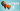# Three Sum - Leet Code Solution

September 13, 2019## Problem Statement

Given an array nums of n integers, are there elements a, b, c in nums such that a + b + c = 0? Find all unique triplets in the array which gives the sum of zero.

Note: The solution set must not contain duplicate triplets.

``````Example:
Given array nums = [-1, 0, 1, 2, -1, -4],

A solution set is:
[
[-1, 0, 1],
[-1, -1, 2]
]``````

## Solution-1

A simple brute force is the start. But, it is so simple. Lets not discuss it. You just need to make sure that result must have unique entries

## Solution-2

Like, in two-sum problem. We used a HashSet. We can use it here as well. Again, to maintain unique elements, it added complexity.

``````public List<List<Integer>> threeSum(int[] nums) {
Set<List<Integer>> result = new HashSet();
int l = nums.length;
for (int i=0; i<l; i++) {
Set<Integer> vals = new HashSet();
for (int j=i+1; j<l; j++) {
if (j == i) continue;

int requiredNum = 0 - (nums[i] + nums[j]);
if (vals.contains(requiredNum)) {
List<Integer> iRes = new ArrayList();

Collections.sort(iRes);
}

if (!vals.contains(nums[j])) {
}
}
}

List<List<Integer>> r = new ArrayList(result);
return r;
}``````

## Solution-3

Lets look at most optimized one.

• Sort the array
• Now, in a loop, start from left and right pointer.
• Keep track of sum. If we found the sum, add it. And, we need to move left and right pointer. One case is where even after moving left and right pointer, the value can be duplicate. Since, we need to avoid duplicates. We need to add a condition here to keep moving untill found a unique element.
• There is a corner case, where handling of duplicate elements has to be handled in outer loop as well. (See line ahead of for loop)
``````public List<List<Integer>> threeSum_2(int[] nums) {
Arrays.sort(nums);
int len = nums.length;

List<List<Integer>> res = new ArrayList<>();
for (int i=0; i<len; i++) {
//to avoid processing duplicate values
if (i > 0 && nums[i-1] == nums[i]) continue;

int l = i+1;
int r = len-1;

while (l < r) {
int s = nums[i] + nums[l] + nums[r];
if (s == 0) {
List<Integer> ir = new ArrayList<>();

l++;
r--;

//to move over duplicate values
while (l < r && nums[l-1] == nums[l]) l++;
while (l < r && nums[r+1] == nums[r]) r--;
}

else if (s < 0) {
l++;
}
else {
r--;
}
}
}
return res;
}``````